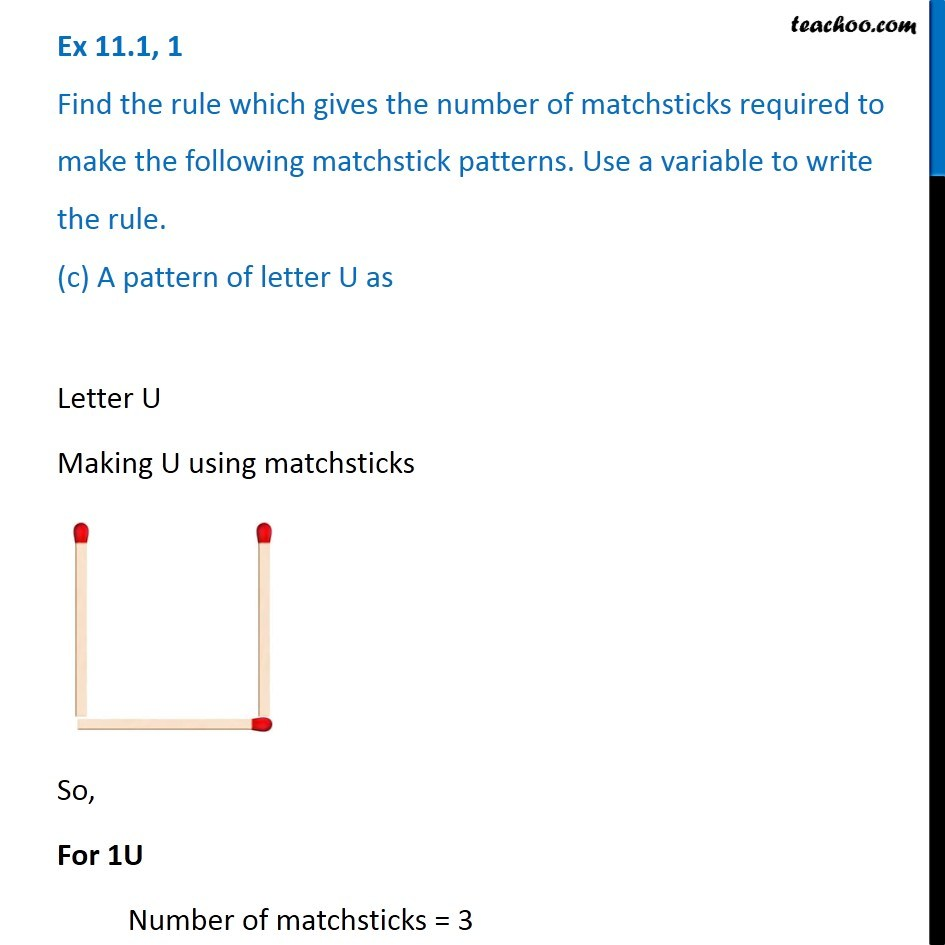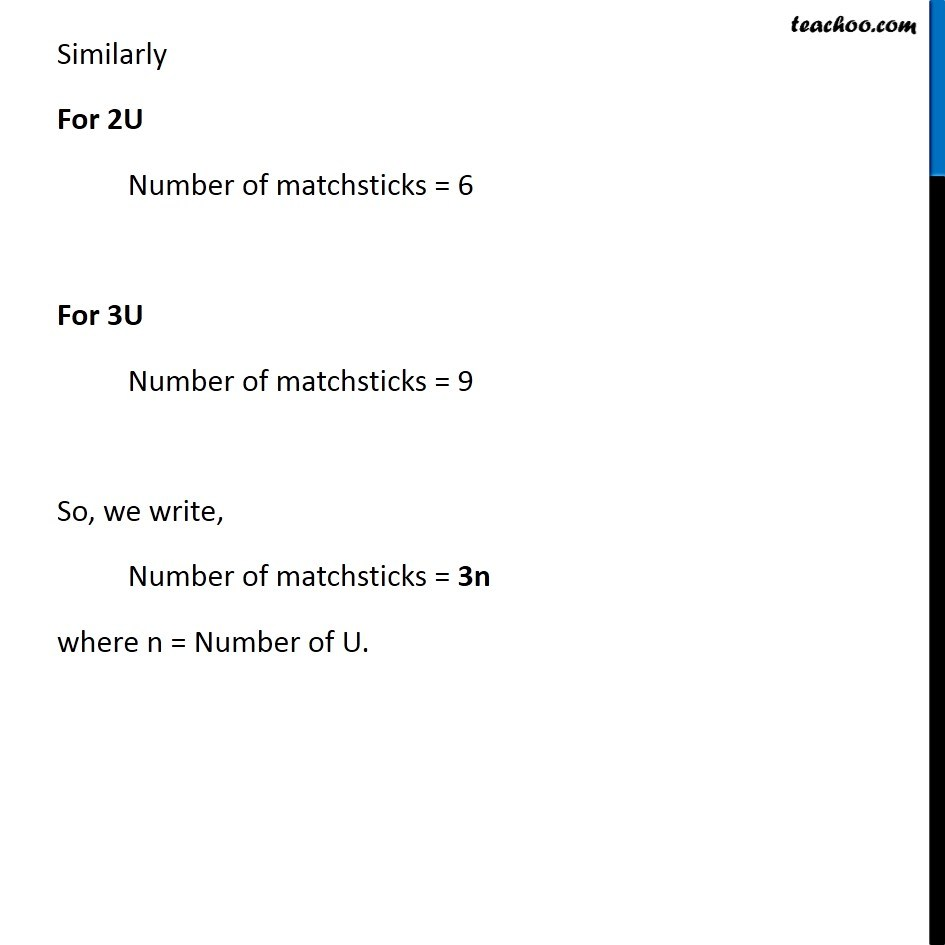Ex 11.1

Chapter 11 Class 6 Algebra
Serial order wiseLearn in your speed, with individual attention - Teachoo Maths 1-on-1 Class

### Transcript

Ex 11.1, 1 Find the rule which gives the number of matchsticks required to make the following matchstick patterns. Use a variable to write the rule. (c) A pattern of letter U asLetter U Making U using matchsticks So, For 1U Number of matchsticks = 3 Similarly For 2U Number of matchsticks = 6 For 3U Number of matchsticks = 9 So, we write, Number of matchsticks = 3n where n = Number of U.